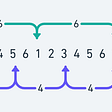# Introduction

In this blog I’d want to describe and show a very famous algorithm, 0–1 Knapsack, but before we do, it’s important to understand what dynamic programming is and why we’re using it here rather than using another technique. In basic terms, dynamic programming is the process of optimizing a recursive algorithm, for understanding it let’s consider an algorithm that calls the same function and supplies it with the same input(s). In this instance, we choose to use dynamic programming to optimize the algorithm. In other words, the primary purpose is to save the solution of subproblems; This is done to prevent re-computation of the same subproblems later on in the algorithm’s iteration. The optimization approach enables us to lower the time complexity of the algorithm from exponential to polynomial, isn’t that amazing!.

# Let’s Understand What is 0–1 Knapsack?

Assume we are given a bag or a knapsack capable of carrying a total weight “W,” as well as a collection of goods or items with their respective weights and costs/profits. Our ultimate objective is to increase the overall number of products in order to maximize earnings while also entirely filling the backpack/knapsack. Now, in terms of coding, examine the weights and values of n elements in order to maximize the total value of the knapsack. To perform this task we take two integer arrays, val[0..n-1] and wt[0..n-1], which are used to represent/denote the values and weights associated with n items. We then proceed to find the greatest value subset of val[] such that the sum of its weights is less than or equal to W, and given an integer W denoting a knapsack’s maximum capacity. Additionally, we have the restriction that we cannot break an object; we must either pick it up entirely or not at all. This is attribute is referred to as the 0–1 property.

# Methods We Can Use

There are 2 methods to tackle the 0–1 knapsack problem;

· Method 1: Using Recursion By Brute Force.

· Method 2: By using Dynamic Programming.

# Approach For Method 1:

The straightforward method is to examine all subgroups of items and determine their combined weight and worth. Consider the subgroups with a total weight less than W. Select the subset with the greatest value from all of these subsets.

Optimal Sub-structure: To consider all subsets of items, there can be two cases for every item.

From here we have 2 cases to check whether our item fits in the Optimal Sub-structure Or Not:

Case 1: The item can be included in the optimal subset.

Case 2: The item cannot be included in the optimal set.

As a result, the greatest value that can be produced from ‘n’ items is equal to the sum of the next two values.

The maximum value that may be engendered with “n-1” items and a weight of W (omitting “nth” item). The value of the “nth” item multiplied by the greatest value procured by the “n-1th” item, and W minus the “nth” item’s weight (including the “nth” item). If the weight of the “nth” item is more sizably voluminous than the weight of the ‘W’ item, the “nth” item cannot be integrated, leaving just Case 1 as our only choice.

# Approach For Method 2:

As with other classic Dynamic Programming (DP) algorithms, re-computation of identical subproblems may be avoided by bottom-up construction of a temporary array DP[][]. The implementation that follows is based on Dynamic Programming.

In dynamic programming, we will investigate the same scenarios as in recursive programming. Consider the columns of a DP[][] table to be all potential weights ranging from ‘1’ to ‘W’, and the rows to be all possible weights. The state DP[i][j] denotes the greatest value of ‘j-weight’ when all values between ‘1 and ith’ are considered. Thus, if we examine ‘wi’ (weight in the ‘ith’ row), we may use it to populate all columns with ‘weight values greater than wi’.

Now, two scenarios are possible:

· Fill in the blank column with ‘wi’.

· Leave ‘wi’ blank in the selected column.

Now we must choose the most favorable of these two alternatives; officially, if we do not fill the ‘ith’ weight in the ‘jth’ column, the DP[i][j] state will be identical to DP[i-1][j], but if we do, DP[i][j] will equal the value of ‘wi’ + the value of the column weighing ‘j-wi’ in the preceding row. Thus, we maximize one of these two options in order to fill the present state.

# Time Complexity Analysis

Time Complexity: O(N*W).
where ‘N’ denotes the number of weight element and ‘W’ is the maximum capacity of our knapsack. As for every weight element we traverse through all weight capacities i.e., the loop will be iterated from 1<=w<=W, where “w ”is the weights of items.

Auxiliary Space: O(N*W).
The use of 2-D array of size ‘N*W’.

--

--

--

## More from Vansh Gupta

Love podcasts or audiobooks? Learn on the go with our new app.

## How to: Create Task Groups Dashboard on Azure DevOps## HostGator review 2021, A detailed review guide## Best Practices for Multi-tenancy in Argo CD## 06. How Objects Behave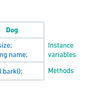## Leetcode: Q198 — House Robber [Easy]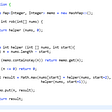## Time to move to the cloud — how to spot fake SaaS from true SaaS!## [Mal Series #15] Android Stealer Cabassous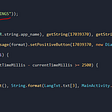## Prims Algorithm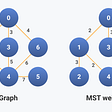## Round-Robin Scheduling Algorithm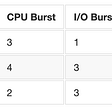## Minimum Spanning Tree & Prim’s Algorithm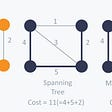## Understanding juggling Algorithm